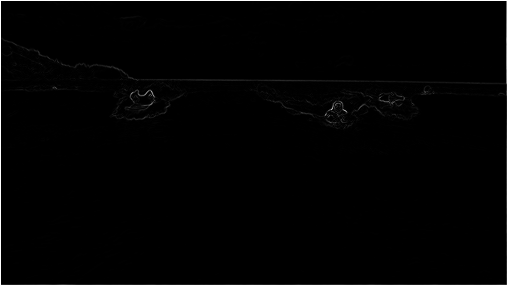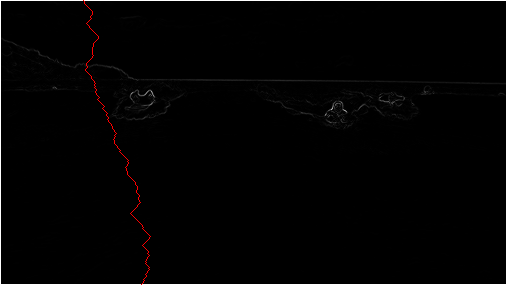## Programming Assignment 7: Seam Carving

Warning: this assignment has not been battle-tested. It is likely that there are more ambiguities and bugs. Please bring those to our attention and we will do our best to clarify and fix.

Seam-carving is a content-aware image resizing technique where the image is reduced in size by one pixel of height (or width) at a time. A vertical seam in an image is a path of pixels connected from the top to the bottom with one pixel in each row. (A horizontal seam is a path of pixels connected from the left to the right with one pixel in each column.) Below left is the original 505-by-287 pixel image; below right is the result after removing 150 vertical seams, resulting in a 30% narrower image. Unlike standard content-agnostic resizing techniques (e.g. cropping and scaling), the most interesting features (aspect ratio, set of objects present, etc.) of the image are preserved.

As you'll soon see, the underlying algorithm is quite simple and elegant. Despite this fact, this technique was not discovered until 2007 by Shai Avidan and Ariel Shamir. It is now a feature in Adobe Photoshop (thanks to a Princeton graduate student), as well as other popular computer graphics applications.In this assignment, you will create a data type that resizes a W-by-H image using the seam-carving technique.

Finding and removing a seam involves three parts and a tiny bit of notation:

1. Notation. In image processing, pixel (x, y) refers to the pixel in column x and row y, with pixel (0, 0) at the upper left corner and pixel (W − 1, H − 1) at the bottom right corner. This is consistent with the Picture data type in stdlib.jar. Warning: this is the opposite of the standard mathematical notation used in linear algebra where (i, j) refers to row i and column j and with Cartesian coordinates where (0, 0) is at the lower left corner.

 (0, 0) (1, 0) (2, 0) (0, 1) (1, 1) (2, 1) (0, 2) (1, 2) (2, 2) (0, 3) (1, 3) (2, 3)

We also assume that the color of a pixel is represented in RGB space, using three integers between 0 and 255. This is consistent with the java.awt.Color data type.

2. Energy calculation. The first step is to calculate the energy of each pixel, which is a measure of the importance of each pixel—the higher the energy, the less likely that the pixel will be included as part of a seam (as we'll see in the next step). In this assignment, you will implement the dual gradient energy function, which is described below. Here is the dual gradient of the surfing image above:The energy is high (white) for pixels in the image where there is a rapid color gradient (such as the boundary between the sea and sky and the boundary between the surfing Josh Hug on the left and the ocean behind him). The seam-carving technique avoids removing such high-energy pixels.

3. Seam identification. The next step is to find a vertical seam of minimum total energy. This is similar to the classic shortest path problem in an edge-weighted digraph except for the following:

• The weights are on the vertices instead of the edges.

• We want to find the shortest path from any of W pixels in the top row to any of the W pixels in the bottom row.

• The digraph is acyclic, where there is a downward edge from pixel (x, y) to pixels (x − 1, y + 1), (x, y + 1), and (x + 1, y + 1), assuming that the coordinates are in the prescribed range.4. Seam removal. The final step is remove from the image all of the pixels along the seam.

The SeamCarver API. Your task is to implement the following mutable data type:

```public class SeamCarver {
public SeamCarver(Picture picture)
public Picture picture()                       // current picture
public     int width()                         // width of current picture
public     int height()                        // height of current picture
public  double energy(int x, int y)            // energy of pixel at column x and row y
public   int[] findHorizontalSeam()            // sequence of indices for horizontal seam
public   int[] findVerticalSeam()              // sequence of indices for vertical seam
public    void removeHorizontalSeam(int[] seam)   // remove horizontal seam from picture
public    void removeVerticalSeam(int[] seam)     // remove vertical seam from picture

public static void main(String[] args)         // unit testing

}
```
• Computing the energy of a pixel. We will use the dual gradient energy function: The energy of pixel (x, y) is Δx2(x, y) + Δy2(x, y), where the square of the x-gradient Δx2(x, y) = Rx(x, y)2 + Gx(x, y)2 + Bx(x, y)2, and where the central differences Rx(x, y), Gx(x, y), and Bx(x, y) are the absolute value in differences of red, green, and blue components between pixel (x + 1, y) and pixel (x − 1, y). The square of the y-gradient Δy2(x, y) is defined in an analogous manner. We define the energy of pixels at the border of the image to use the same pixels but to replace the non-existant pixel with the pixel from the opposite edge. 2552 + 2552 + 2552 = 195075.

As an example, consider the 3-by-4 image with RGB values (each component is an integer between 0 and 255) as shown in the table below.

 (255, 101, 51) (255, 101, 153) (255, 101, 255) (255,153,51) (255,153,153) (255,153,255) (255,203,51) (255,204,153) (255,205,255) (255,255,51) (255,255,153) (255,255,255)

The ten border pixels have energy 195075. Only the pixels (1, 1) and (1, 2) are nontrivial. We calculate the energy of pixel (1, 2) in detail:

Rx(1, 2) = 255 − 255 = 0,
Gx(1, 2) = 205 − 203 = 2,
Bx(1, 2) = 255 − 51 = 204,
yielding Δx2(1, 2) = 22 + 2042 = 41620.

Ry(1, 2) = 255 − 255 = 0,
Gy(1, 2) = 255 − 153 = 102,
By(1, 2) = 153 − 153 = 0,
yielding Δy2(1, 2) = 1022 = 10404.

Thus, the energy of pixel (1, 2) is 41620 + 10404 = 52024. Similarly, the energy of pixel (1, 1) is 2042 + 1032 = 52225.

We calculate the energy of the border pixel (1, 0) in detail:

Rx(1, 0) = 255 − 255 = 0,
Gx(1, 0) = 101 − 101 = 0,
Bx(1, 0) = 255 − 51 = 204,
yielding Δx2(1, 0) = 2042 = 41616.

Since there is no pixel (x, y - 1) we calculate between pixel (x, y + 1) and pixel (x, height − 1).
Ry(1, 0) = 255 − 255 = 0,
Gy(1, 0) = 255 − 153 = 102,
By(1, 0) = 153 − 153 = 0,
yielding Δy2(1, 2) = 1022 = 10404.

Thus, the energy of pixel (1, 2) is 41616 + 10404 = 52020.

Remove this line: The table below has been modified:

 20808 52020 20808 20808 52225 21220 20809 52024 20809 20808 52225 21220

• Finding a vertical seam. The findVerticalSeam() method should return an array of length H such that entry x is the column number of the pixel to be removed from row x of the image. For example, consider the 6-by-5 image below (supplied as 6x5.png).

 ( 97, 82,107) (220,172,141) (243, 71,205) (129,173,222) (225, 40,209) ( 66,109,219) (181, 78, 68) ( 15, 28,216) (245,150,150) (177,100,167) (205,205,177) (147, 58, 99) (196,224, 21) (166,217,190) (128,120,162) (104, 59,110) ( 49,148,137) (192,101, 89) ( 83,143,103) (110, 79,247) (106, 71,174) ( 92,240,205) (129, 56,146) (121,111,147) ( 82,157,137) ( 92,110,129) (183,107, 80) ( 89, 24,217) (207, 69, 32) (156,112, 31)

The corresponding pixel energies are shown below, with a minimum energy vertical seam highlighted in red. In this case, the method findVerticalSeam() should return the array { 3, 3, 3, 3, 3}. (The yellow is just to make the table easier to read.)

Remove: table has changed.

 54572 51263 25436 17321 47599 36173 69374 23346 51304 31519 55112 61426 39387 47908 61346 35919 38887 46630 42086 31400 37927 14437 63076 16315 17637 47935 34879 10471 60270 42607

When there are multiple vertical seams with minimal total energy, your method can return any such seam.

• Finding a horizontal seam. The behavior of findHorizontalSeam() as analogous to that of findVerticalSeam() except that it should return an array of W such that entry y is the row number of the pixel to be removed from column y of the image.

• Performance requirements. The width(), height(), and energy() methods should take constant time in the worst case. All other methods should run in time at most proportional to W H in the worst case. For faster performance, do not construct an explicit EdgeWeightedDigraph object.

• Exceptions. Your code should throw an exception when called with invalid arguments.

• By convention, the indices x and y are integers between 0 and W − 1 and between 0 and H − 1 respectively. Throw a java.lang.IndexOutOfBoundsException if either x or y is outside its prescribed range.

• Throw a java.lang.IllegalArgumentException if removeVerticalSeam() or removeHorizontalSeam() is called with an array of the wrong length or if the array is not a valid seam (i.e., two consecutive entries differ by more than 1).

Picture class will throw this exception. Throw a java.lang.IllegalArgumentException if either removeVerticalSeam() or removeHorizontalSeam() is called when the width or height is 1.

Analysis of running time.  Analyze your approach to this problem giving estimates of its time and space requirements by answering the relevant questions in the readme.txt file.

• Estimate empirically the running time (in seconds) to remove one row and one column from a W-by-H image as a function of W, and H. Use tilde notation to simplify your answer.

Extra Credit. Submit an image (either your own, or one in the public domain) for which the technique works particularly well (or poorly). Include a brief description in the readme of what makes the image particularly (un)amenable to the technique.

Challenge for the bored.

The challenges worth extra credit are quite difficult, and you certainly shouldn't attempt them just for the points. Make sure to note if you've successfully completed them in your readme.

1. Your energy() method implemented the dual gradient energy function. Try out other energy functions. No extra credit.

2. Optimize multiple calls to the find seam and remove seam methods by caching the previous call's information. One point of extra credit if you can get the algorithm running at interactive speeds for small images (say 200 x 200).

3. Implement an interactive object-removal feature: The user highlights an area of the image, and that portion of the image is forced to zero energy. Rows and columns are then successively removed until every pixel in that zero-energy region has been removed. One point of extra credit.

Submission.  Submit SeamCarver.java, and any other files needed by your program (excluding those in stdlib.jar and algs4.jar). Finally, submit a readme.txt file and answer the questions.

This assignment was developed by Josh Hug, Maia Ginsburg, and Kevin Wayne.